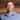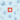TechnologyOct 11, 2016

# Solving Logical Business Problems Using Prolog: Part TwoMicah JonesIn part one of this series, we were introduced to the Prolog language. In this part, we will look at an extremely useful programming tool called Constraint Logic Programming (CLP).

In CLP, we declaratively provide a set of logical and/or arithmetic constraints, and then let a solving engine infer all possible values that fit those constraints. Intuitively, we may think of CLP as a solution to satisfiability problems, generalized to include both boolean and arithmetic variations. Many logically complex real-world problems (such as the scheduling example we will discuss in part three) can be reduced to concise, solvable CLP constraints.

For the following examples, we will be using the CLP(FD) libraries in SWI-Prolog. You can include those libraries with the following command:

use_module(library(clpfd)).

We will look at examples of the two most general constraint types: arithmetic and boolean.

## Arithmetic Constraints

You might think of arithmetic CLP as being akin to linear algebra, in which the constraints are a system of linear equations and/or inequalities.

The arithmetic constraint syntax for clpfd is as follows:

Expr1 #= Expr2

Expr1 equals Expr2

Expr1 #\= Expr2

Expr1 is not equal to Expr2

Expr1 #>= Expr2

Expr1 is greater than or equal to Expr2

Expr1 #=< Expr2

Expr1 is less than or equal to Expr2

Expr1 #> Expr2

Expr1 is greater than Expr2

Expr1 #< Expr2

Expr1 is less than Expr2

At the most simple level, you can use CLP for variable assignment:

?- X #= 2.

X = 2.

CLP can also infer values from more complex expressions:

?- 2*X #= 10.

X = 5.

If a specific value cannot be inferred for a variable, we get a domain instead:

?- X #> 3.

X in 4..sup.

?- 3 #> X.

X in inf..2.

Note that the clpfd libraries use sup to denote infinity and inf to denote negative infinity. Therefore, the first solution above indicates that the integer X is in the domain between 4 and infinity, inclusive. We can add a constraint to narrow that domain using an in expression as follows:

?- X #> 3, X in 0..10.

X in 4..10.

CLP can also be used to solve an entire system of arithmetic constraints with multiple variables:

?- 3*X - 4*Y #= 16, X + Y #= 10, [X,Y] ins 0..sup.

X = 8,

Y = 2.

The ins operator above is similar to in, but it simultaneously sets domains for multiple variables in a list. In this case, we confine the domain for X and Y to the set of positive integers so the solving engine can infer a single solution.

## Boolean Constraints

As mentioned earlier, CLP can be used to model and solve boolean satisfiability problems. That is, given a boolean expression, we want to find a combination of variable assignments such that the expression evaluates to true.

The boolean constraint syntax for clpfd is as follows:

#\ Q

True iff Q is false

P #\/ Q

True iff either P or Q

P #/\ Q

True iff both P and Q

P #\ Q

True iff either P or Q, but not both

P #<==> Q

True iff P and Q are equivalent

P #==> Q

True iff P implies Q

P #<== Q

True iff Q implies P

Using this syntax, basic boolean expressions are easy to construct and solve:

?- X #/\ Y.

X = Y, Y = 1.

A “true” is represented by 1 and a “false” by 0. Therefore, you can read the above solution as “X must be equal to Y, which is equal to true,” which is equivalent to saying that both X and Y must be true.

When multiple solutions are valid, clpfd will not automatically bind values to variables. However, there are times when we will want to know at least one specific solution. In those cases the label predicate is useful for non-deterministic assignment of satisfying values:

?- X #\/ Y, label([X,Y]).

X = 0,

Y = 1 ;

X = 1,

Y = 0 ;

X = Y, Y = 1.

Here, there are three possible solutions to satisfy “X or Y”: X is false and Y is true; X is true and Y is false; or X and Y are both true.

You can also conjunctively combine expressions using Prolog’s comma operator (,):

?- X #\/ (Y #/\ #\Z), X #/\ Z, label([X,Y,Z]).

X = Z, Z = 1,

Y = 0 ;

X = Y, Y = Z, Z = 1.

In fact, you can write multiple constraint expressions using the same variables in different parts of a program’s control flow, even in separate predicates, only calling label to assign satisfying values to those variables when you’re done. This allows for very complex satisfiability problems to be broken down into manageable chunks. We will do exactly that in part three.

## Conclusion

Constraint Logic Programming is one of the most powerful tools available to the Prolog programmer. As stated in part one, Prolog is useful for exploring a search space, and CLP allows us define those search spaces in terms of complex arithmetic and boolean expressions.

Using CLP effectively to solve real-world problems does require some creativity, as we must reduce those problems to constraints. A major application of CLP is in the realm of resource allocation problems. We will handle one such problem in part three, when we build an employee scheduling system with several domain-specific rules.

Micah Jones is a consultant in Credera’s Integration & Data Services (IDS) practice. In 2011, he received his doctorate in computer science from the University of Texas at Dallas, specializing in programming language-based security.